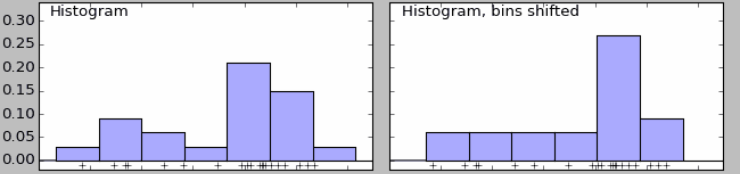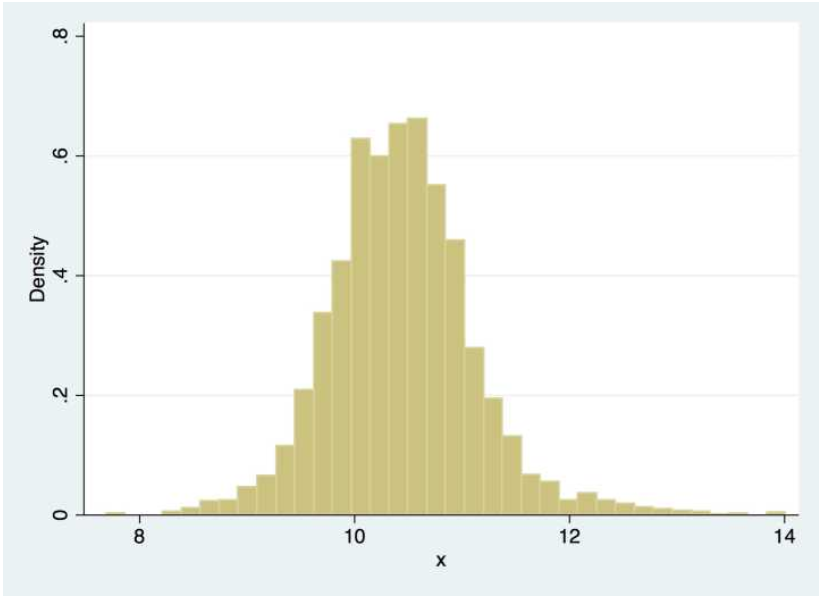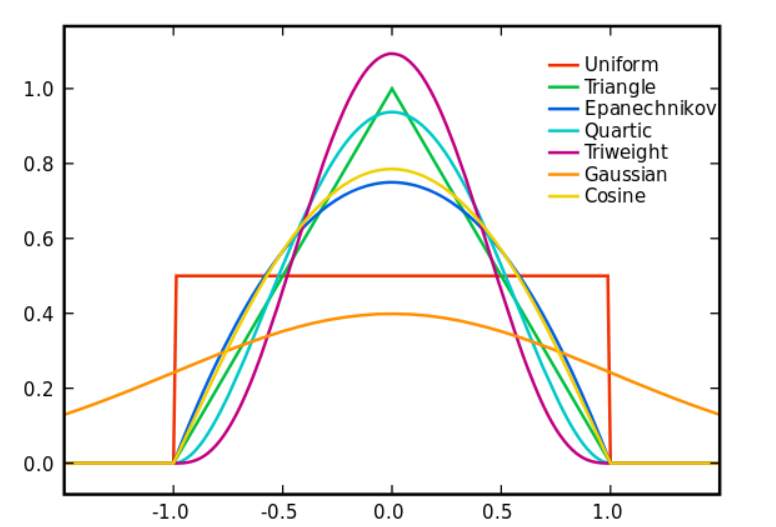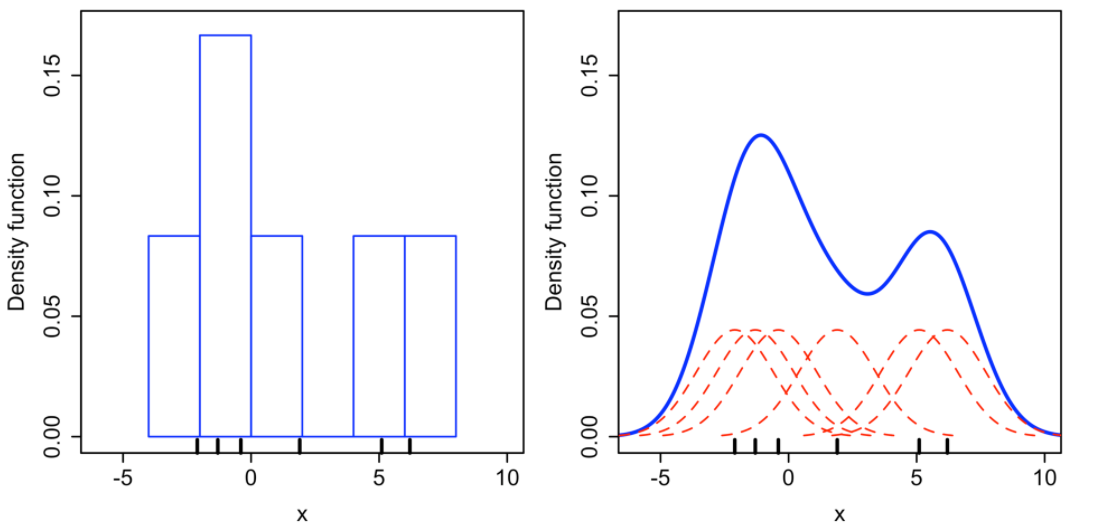1.参数估计方法

2.非参数估计

# 直方图到核密度估计f^(x)=12hlimh>0Nxi[xh,x+h]Ntotal
Nxi[xh,x+h]$N_{xi \in [x-h,x+h]}$时该邻域中的样本点数量，Ntotal$N_{total}$样本集的总数量，最后对该邻域内的密度值取平均便得到x$x$点的密度函数值f(x)$f(x)$。把上面的式子进行改写：
f^(x)=12hNtotali=xhx+hxi=1hNtotali|xxi|2h<1,h>0

K(x)=121{x<1}$K(x)={1 \over 2}1\{x<1\}$，那么：
f^(x)=1hNtotaliK(|xxi|h)

f^(x)=1hNtotaliK(|xxi|hdx=1NtotaliK(t)dt=K(t)dt

### 核函数### 带宽的选择

MISE(h)=E(f^(x)f(x))2dx

AMISE(h)=R(K)nh+14m2(K)2h4R(f′′)

R(K)=K(x)2dx,m2(K)=x2K(x)dx

hAMISE(h)=R(k)nh2+m2(K)2h3R(f′′)=0

hAMISE=R(K)15m2(K)25R(f′′)15n15

# 总结

### 参考

©️2019 CSDN 皮肤主题: 大白 设计师: CSDN官方博客